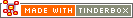^sum( group , attribute[, precision] )^Code Type: Calculation Code Scope of Action: group

^sum( group , attribute[, precision] )^

Returns the sum of the attribute values for all the notes within the group.

An optional third argument lets you set the number of decimal points that are exported by the numeric group markup elements(^sum^, ^min^, ^max^, ^mean^, ^count^). Note that the precision is always the third argument.

Internally, the sum() operator may be used.

 Up: Group-based Next: ^sectionCloud( [item, count] )^

[Last updated: 14 Dec 2009, using v5.0]